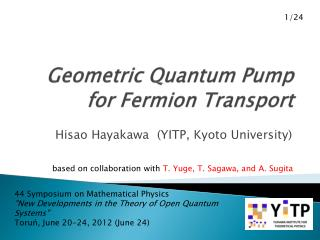# Geometric Quantum Pump for Fermion Transport - PowerPoint PPT PresentationDownload PresentationGeometric Quantum Pump for Fermion Transport

Geometric Quantum Pump for Fermion TransportDownload Presentation## Geometric Quantum Pump for Fermion Transport

- - - - - - - - - - - - - - - - - - - - - - - - - - - E N D - - - - - - - - - - - - - - - - - - - - - - - - - - -
##### Presentation Transcript

1. 1/24 Geometric Quantum Pump for Fermion Transport Hisao Hayakawa(YITP, Kyoto University) based on collaboration with T. Yuge,T. Sagawa, and A. Sugita 44 Symposium on Mathematical Physics "New Developments in the Theory of Open Quantum Systems" Toruń, June 20-24, 2012 (June 24)

2. Collaborators Takahiro Sagawa (YITP) TetsuroYuge (YITP->Osaka Univ.) Ayumu Sugita (Osaka City Univ.) & Ryosuke Yoshii (YITP) I would like to thank all these collaborators on this problem. 2/24

3. Contents • Introduction • Geometric Pump for Fermion Transport • Setup • Main Results • Special Cases & Example • Application to Entropy Production • Discussion • Conclusion 3/24

4. Contents • Introduction • Geometric Pump for Fermion Transport • Setup • Main Results • Special Cases & Example • Application to Entropy Production • DIscussion • Conclusion 4/24

5. Introduction •  In mesoscopic systems, a current can exist even at zero bias. This effect is called the quantum pumping. Nano-machine to extract work 5/24

6. 6/24 Previous studies and question • Adiabatic quantum pump • Geometric effect is important (P. W. Brower, PRB58, 10135 (1998)). • Control of system parameters • Can we get the pump effect by controlling reservoir parameters?

7. Contents • Introduction • Geometric Pump for Fermion Transport • Setup • Main Results • Special Cases & Example • Application to Entropy Production • Discussion • Conclusion 7/24

8. Full counting statistics(FCS) 8/24 • Projection measurement • Counting: • Number of spinless electrons transfer from L to R • Statistics & cumulant generating function

9. 9/24 How can we compute FCS? • We assume that the total Hamilitonian satisfies von-Neumann equation. • We calculate the modified von-Neumann equation via the counting field: • Ref.

10. Quantum Master equation χ 10/24

11. Adiabatic modulation 11/24 Control parameters

12. 12/24 Net current in an adiabatic cycle where

13. N non-interacting quantum dots • Based on FCS Born-Markov approximation + rotational wave approximation (RWA), we obtain 13/24

14. Contents • Introduction • Geometric Pump for Fermion Transport • Setup • Main Results • Special Cases & Example • Application to Entropy Production • Discussion • Conclusion 17/24

15. Application to entropy production • The method we adopted can be used for the calculation of any other quantities. • We can discuss the path dependence of the nonequilibrium entropy production. • Namely, the entropy is a geometric quantity under a nonequilibrium situation. • Note that the entropy production is a non-conserved quantity. • See Sagawa and HH, PRE 84, 051110 (2011). heat 18/24

16. Results for entropy production parameters space Path-dependence quasi-static process 19/24

17. Contents • Introduction • Geometric Pump for Fermion Transport • Setup • Main Results • Special Cases & Example • Application to Entropy Production • Discussion • Conclusion 20/24

18. Discussion 1 • Effects of spins and many-body interactions • We have already calculated Kondo problem (R. Yoshii and HH, in preparation). • The many-body effect can be absorbed via Schrieffer-Wolff transform. • Without the potential scattering term, the result is unchanged. • If we introduce the term, the symmetry of evolution matrix is changed. So there is possibility to have the geometric effect. 21/24

19. Discussion 2 ; Effect of statistics • So far, we assume that particles are Fermions. • However, our analysis is based on RWA (quasi-classical) and the result contains only distribution function of reservoirs. • We expect that the geometric effect can appear for Bosons. • See JieRen et al., PRL 104, 170601 (2010). 22/24

20. Contents • Introduction • Geometric Pump for Fermion Transport • Setup • Main Results • Special Cases & Example • Application to Entropy Production • Discussion • Conclusion 23/24

21. Summary • We have analyzed a quantum pump effect on Fermion transport. • We have found that spinless Fermions without interactions do not have any geometric effect if we control reservoir parameters. • We confirm that there exist geometric effects for the control of system parameters. • Such an idea can be used for entropy production. • Geometric effects are important. • We are now calculating the Kondo problem. 24/24

22. Thank you for your attention.